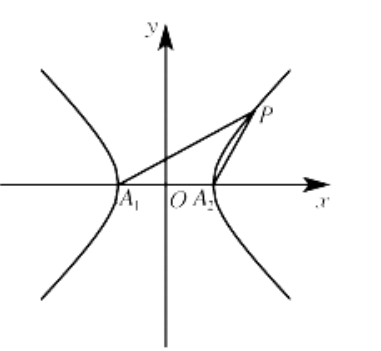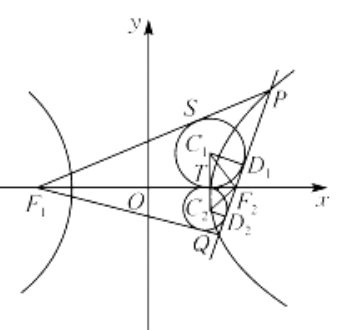$\text{A.}$ 若 $\angle F_1 P F_2=\frac{\pi}{3}$, 则 $\triangle F_1 P F_2$ 的面积为 $\frac{2 \sqrt{3}}{3}$ $\text{B.}$ 记直线 $P A_1$ 与直线 $P A_2$ 的斜率分別为 $k_1, k_2$, 则 $k_1 k_2=1$ $\text{C.}$ 若 $\angle A_1 P A_2=2 \angle P \Lambda_1 \Lambda_2$, 则 $\angle P A_1 A_2=\frac{\pi}{8}$ $\text{D.}$ 延长 $P F_2$ 交 $C$ 的右支于点 $Q$, 设 $\triangle P F_1 F_2$ 与 $\triangle Q F_1 F_2$ 的内切圆半径分別为 $r_1, r_2$, 则 $r_1 \cdot r_2=6-4 \sqrt{2}$
【答案】 BCD

【解析】 设 $\left|P F_1\right|=m,\left|P F_2\right|=n$, 所以 $m-n=2 \sqrt{2}$, 由余弦定理得 $\left|F_1 F_2\right|^2=\left|P F_1\right|^2+\left|P F_2\right|^2-2\left|P F_1\right| \cdot$ $\left|P F_2\right| \cos \angle F_1 P F_2$, 即 $16=m^2+n^2-m n$. 所以 $16=m^2+n^2-m n=(m-n)^2+m n=8+m n$. 所以 $m n=8$. 所 以 $\triangle F_1 P F_2$ 的面积 $S=\frac{1}{2} m n \sin \angle F_1 P F_2=2 \sqrt{3}$, 故 $\mathrm{A}$ 错误; 由题意知, $A_1(-\sqrt{2}, 0), A_2(\sqrt{2}, 0), F_1(-2,0)$, $F_2(2,0)$, 设 $P\left(x_0, y_0\right)$, 所以 $\frac{x_0^2}{2}-\frac{y_0^2}{2}=1$, 即 $y_0^2=x_0^2-2$. 所以 $k_1=\frac{y_b}{x_0+\sqrt{2}}, k_2=\frac{y_0}{x_0-\sqrt{2}}$. 所以 $k_1 k_2=\frac{y_b}{x_0+\sqrt{2}} \cdot$ $\frac{y_0}{x_0-\sqrt{2}}=\frac{y_0^2}{x_0^2-2}=1$, 故 B 正确;